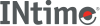﻿ remainderINtime SDK Help
remainder

Returns the floating-point remainder `r = x - ny` when `y` is non-zero. The value `n` is the integral value nearest the exact value `x/y`. When `|n-x/y| = 1/2`, the value `n` is chosen to be even.

```#include <math.h>

double remainder (double x, double y);
```

#### Parameters

`x`, `y`
Any numeric expression.

#### Return Values

Upon successful completion, these functions shall return the floating-point remainder `r = x - ny` when `y` is non-zero.

If `x` or `y` is NaN, a NaN shall be returned.

If `x` is infinite or `y` is 0 and the other is non-NaN, a domain error shall occur, and either a NaN (if supported), or an implementation-defined value shall be returned.

#### Requirements

Versions Defined in Include Link to
INtime 3.0 intime/rt/include/math.h math.h clib.lib